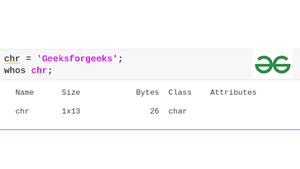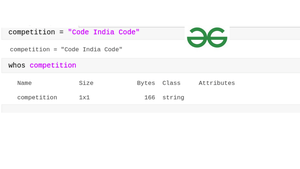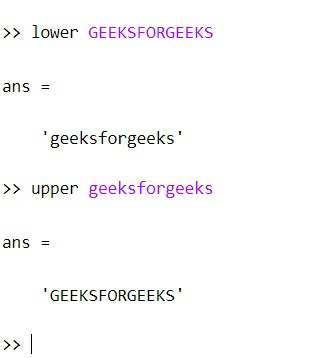# Characters and Strings in MATLAB

• Difficulty Level : Expert
• Last Updated : 15 Mar, 2022

In this article, we shall see how to deal with characters and Strings in MATLAB. A data type is an attribute/keyword that specifies the type of data that the object can hold: numeric data or text data. By default, MATLAB stores all numeric variables as double-precision floating-point values. Additional data types store text, integer or single-precision values, or a combination of related data in a single variable.

The text data is stored in a character array and String array. We shall the use case of both character and string in MATLAB:

### Character:

Just like in C/C++/Java, a character is a data type that stores single character data within single quotes. In Matlab, you store the entire text within single quotes and it will be treated as a character.

Example 1:

## Matlab

 `% Character is enclosed within single quotes` `chr = ``'Geeksforgeeks'` `whose chr`

Output:The text ‘Geeksforgeeks’ is 13 characters long, and chr stores it as a 1-by-13 character vector. As in the above image you can notice that the chr variable belongs to the Char class i.e., character.

If the text includes single quotes, use two single quotes within the definition.

Example 2:

## Matlab

 `% MATLAB code for Single Quote` `% Sentence Inside Character` `chr = ``'Geeksforgeeks is hosting, '` `'Geeks Premier League'``' for all its writers.'``;`

Output:

`chr = 'Geeksforgeeks is hosting, ''Geeks Premier League'' for all its writers.'`

### Character Operation in MATLAB:

• Indexing: The index is used to select the subset of the text from the sequence of characters. To perform the index operation in Matlab the index should be enclosed within parenthesis().

Example 3:

## Matlab

 `% MATLAB code for indexing` `practice = ``'Geeksforgeeks'``;` `practice(1:5)`

Output:

`ans='Geeks'`

Example 4:

## Matlab

 `% MATLAB code for indexing` `practice(1)`

Output:

`ans='G'`
• Concatenate: Concatenate character vector with square brackets, merges two different characters in one.

Example 5:

## Matlab

 `% MATLAB code for Concatenate` `str1 = ``'Geeks'``;` `str2 = ``'Premier League'``;` `str3 = ``' 2022'``;` `contest = [str1,str2,str3]`

Output:

`contest = 'GeeksPremier League 2022'`
• append: append function inserts a new character to the existing character. The functionality of concatenating is the same but the append function is recommended because it treats string arrays, character vectors, and cell arrays of character vectors consistently.

Example 6:

## Matlab

 `% MATLAB code for append` `com = ``'Geeks'``;` `contest = append(com,``'Premier League'``);` `display(contest)`

Output:

`contest = 'GeeksPremier League'`

## String

String arrays provide a set of functions for working with text as data, i.e., it is a sequence of characters that is enclosed within double-quotes.

Example 7:

## Matlab

 `% MATLAB code for string with whose` `competition = ``"Code India Code"` `whose competition`

Output:### String Operations:

• strlength: Lengths of strings return the total number of characters in a given string.

Example 8:

## Matlab

 `% MATLAB code for get a string length` `str = ``"Geeks Premier League"``;` `num = strlength(str)`

Output:

`num=20`
• isstring: Checks if the given string is string or not. Returns 0 if it is not a string and 1 if is a string.

Example 9:

## Matlab

 `% MATLAB code for isstring` `str = isstring(``'Mathworks'``)`

Output:

`str = 0`

0 because it is false, the entered value is in Character(enclosed within single quotes).

Example 10:

## Matlab

 `% MATLAB code for isstring ` `str = isstring(``"Mathworks"``)`

Output:

`1`
• replace: When you have a string and want to update the old content to new then replace function is used. Using replace function you can modify the old string data to the new data within the same variable.

Example 11:

## Matlab

 `change = ``"Coding is tough"``;` `str = replace(change,``"tough"``,``"easy"``);` `display(``"Before:"` `+change)` `display(``"After:"` `+str)`

Output:

```"Before: Coding is tough"
"After:Coding is easy"```
• lower and upper: lower and upper functions are used to modify the given string. lower convert strings to lowercase whereas upper convert strings to uppercase.

## Matlab

 `% MATLAB Code for String Lower and Upper` `lower GEEKSFORGEEKS` `upper geeksforgeeks`

Output:• reverse: The reverse function is used to return the same string but in reverse order.

Example 12:

## Matlab

 `str1 = reverse(``"Matlab is fun"``)`

Output:

`str= "nuf si baltaM"`
• strcmp: When we have two different strings Matlab even allows us to compare these two strings. Using strcmp, you can compare strings. If it is equal it will return 1 and if is not equal it will return 0.

Note: Comparing is case-sensitive.

Example 13:

## Matlab

 `str1 = ``'Geeksfor'``;` `str2 = ``'forGeeks'``;` `cmp = strcmp(str1,str2)`

Output:

`0`

Example 14:

## Matlab

 `str1 = ``'Geeks'``;` `str2 = ``'Geeks'``;` `cmp = strcmp(str1,str2)`

Output:

`1`

My Personal Notes arrow_drop_up
Recommended Articles
Page :